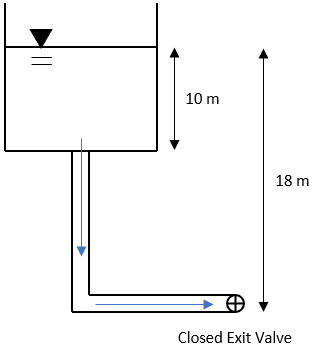## Water Tower

Consider a small city’s water tower has its storage tank open to the atmosphere, and a 1 m^2 diameter connector pipe is attached. What is the gauge pressure at the outlet if the exit valve is closed? Assume the pipe and tank are completely full of water.Hint
Note the density of water is $$1,000\:kg/m^3$$ .
Hint 2
The pressure field in a static liquid:
$$P=\rho gh=\gamma h$$$where $$\rho$$ is the density, $$g$$ is the acceleration due to gravity, $$h$$ is the height, and $$\gamma$$ is the fluid’s specific weight. The pressure field in a static liquid: $$P=\rho gh=\gamma h$$$
where $$\rho$$ is the density, $$g$$ is the acceleration due to gravity, $$h$$ is the height, and $$\gamma$$ is the fluid’s specific weight. Since the density of water is $$1,000\:kg/m^3$$ :
$$P=(1,000\frac{kg}{m^3})(9.8\frac{m}{s^2})(18m)=176,400\frac{kg}{m\cdot s^2}=176.4\:kPa$$$Alternatively, we could solve for pressure using the specific weight of water: $$P=(9,800\frac{kg}{m^2\cdot s^2})(18m)=176,400\frac{kg}{m\cdot s^2}=176.4\:kPa$$$
176.4 kPa